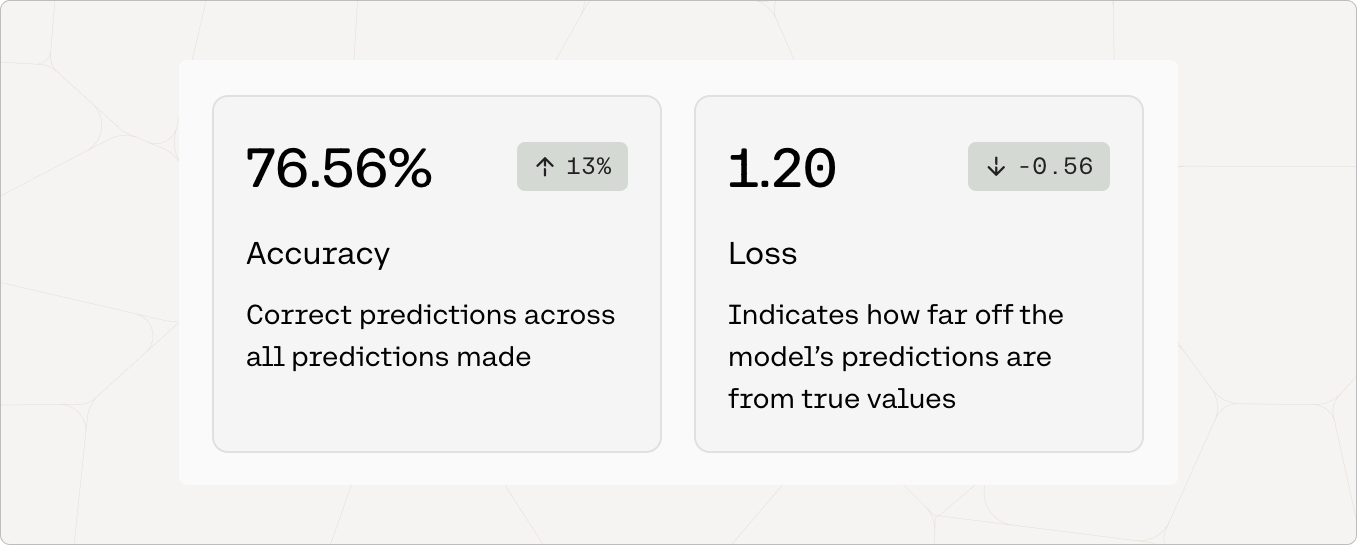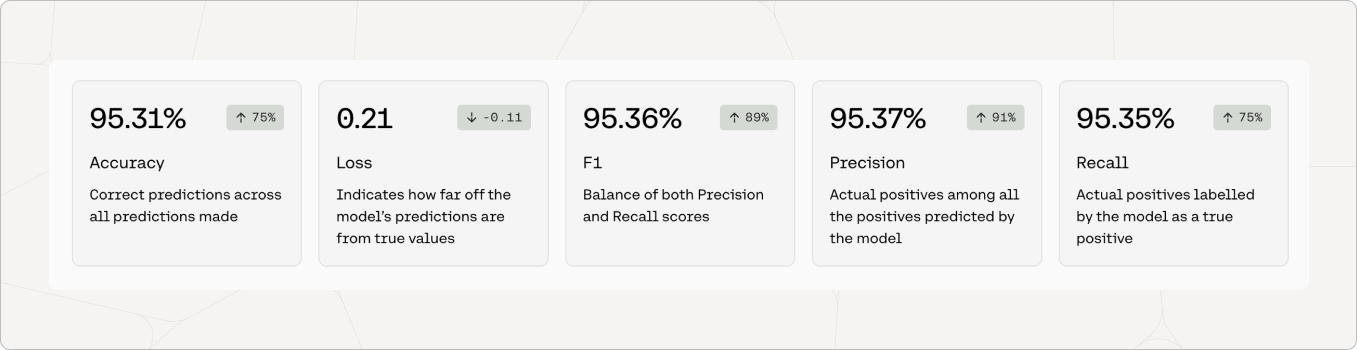# Custom Model Metrics

When you train a custom model, we provide you some measures to see how well your model might perform at the task provided.

# Generate Model Metrics

Please note that Generate model outputs are often best evaluated qualitatively, so the performance metrics provided alone will not provide a comprehensive understanding of the model’s performance.

When you train a Generate custom model, you will see metrics that look like this:## Accuracy

Accuracy is a measure of how many predictions the model made correctly out of all the predictions in an evaluation. To evaluate Generate models for accuracy, we ask it to predict certain words in the user uploaded data.

The number in the pill (eg. 13%) is the difference between the accuracy of the default model when the user started training, and the accuracy of the model that is deployed. This difference is a proxy for how much accuracy improvement was gained by training the model on the dataset.

## Loss

Loss is a measure that describes how bad or wrong a prediction is. Accuracy may tell you how many predictions the model got wrong, but it will not describe how incorrect the wrong predictions are. If every prediction is perfect, the loss will be 0.

To evaluate Generate models for loss, we ask the model to predict certain words in the user provided data and evaluate how wrong the incorrect predictions are. A loss around 11 indicates totally random performance.

For this reason, the loss should decrease as the model improves. The number in the pill (e.g -0.56) is the difference between the loss when the default model started training and when it was deployed. This difference is a proxy for how much loss improvement was gained by training the model on your dataset.

# Classify and Embed Model Metrics

Classify and Embed custom Models are both trained using data of examples mapping to predicted labels, and for that reason they are evaluated using the same methods and performance metrics. You can also provide a test set of data that we will use to calculate performance metrics. If a test set is not provided, we will split your training data randomly to calculate performance metrics.

When you train Classify and Embed custom models, you will see metrics that look like this:## Accuracy

Accuracy is a measure of how many predictions the model made correctly out of all the predictions in an evaluation. To evaluate Embed and Classify models for accuracy, we ask the model to predict labels for the examples in the test set. In this case, the model predicted 95.31% of the labels correctly.

The number in the pill (eg. 75%) is the difference between the accuracy of the default model when the user started training, and the accuracy of the model that is deployed. This difference is a proxy for how much accuracy improvement was gained by training the model on the dataset.

## Loss

Loss is a measure that describes how bad or wrong a prediction is. Accuracy may tell you how many predictions the model got wrong, but it will not describe how incorrect the wrong predictions are. If every prediction is perfect, the loss will be 0.

To evaluate Classify and Embed models for loss, we ask the model to predict labels for the examples in the test set and evaluate how wrong the incorrect predictions are.

For this reason, the loss should decrease as the model improves. The number in the pill (e.g -0.11) is the difference between the loss when the default model started training and when it was deployed. This difference is a proxy for how much loss improvement was gained by training the default model on your dataset.

## Precision

Precision is a measure that shows, for a given label, how correct the model was when it predicted the label. It’s calculated by taking the number of true positives and dividing it by the sum of true positives and false positives.

For example, let’s say we have a test set of 100 examples. 50 of them are label `A` and 50 of them are label `B`. If the model guessed label `A` for every prediction (100 times), every incorrectly predicted label `B` would be a false positive. The precision of label `A` would be 50%.

This is calculated for every label. The number shown in the metrics are the macro-weighted average of the precision across labels.

## Recall

Recall is a measure that shows how often the model predicted a given label correctly. It’s calculated by taking the number of true positives and dividing it by the sum of true positives and false negatives.

For example, let’s say we have a test set of 100 examples. 50 of them are label `A` and 50 of them are label `B`. If the model guessed label `A` for every prediction (100 times), there would be no false negative predictions of label `A`. The recall of label `A` would be 100%.

This is calculated for every label. The number shown in the metrics are the macro-weighted average of the recall across labels.

## F1

Optimizing for either precision or recall often means sacrificing quality in the other. In the example above, 100% recall for label `A` does not mean that it was a great model, as evidenced by the precision. The F1 score attempts to provide a balanced measure of performance between precision and recall.

The number shown in the metrics are the macro-weighted average of F1 across labels.

For Recall, Precision, and F1, the number in the pill is a proxy for how much improvement was gained by training the default model on your dataset.

You can see the detailed calculations to evaluate Embed and Classify models in this blog post.